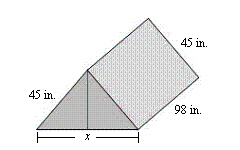Name:    Quiz 6.1-6.3

Multiple Choice
Identify the choice that best completes the statement or answers the question.

Find the surface area of the figure.

1.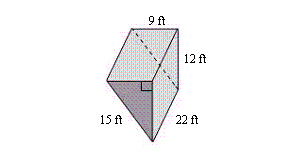a. 900 ftc. 1008 ftb. 1692 ftd. 896 ft2.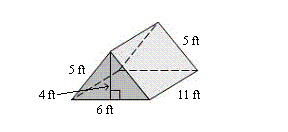a. 188 ftc. 224 ftb. 200 ftd. 218 ftFind the surface area of the figure.

3.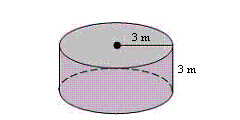a. 84.78 mc. 113.04 mb. 136.04 md. 75.36 m4.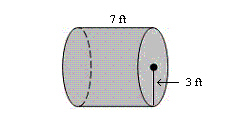a. 75.36 ftc. 160.14 ftb. 452.16 ftd. 188.4 ft5.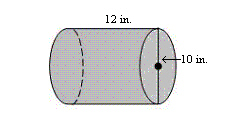a. 1004.8 in.c. 816.4 in.b. 533.8 in.d. 910.6 in.Find the lateral surface area of the cylinder.  Round your answer to the nearest tenth.

6.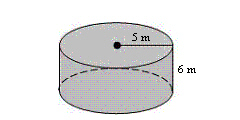a. 94.2 mc. 199.4 mb. 157 md. 188.4 m7.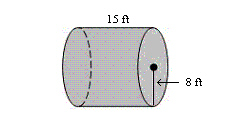a. 753.6 ftc. 376.8 ftb. 401.9 ftd. 767.6 ft8.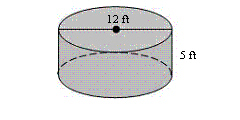a. 226.1 ftc. 376.8 ftb. 188.4 ftd. 94.2 ftNumeric Response

1.

The back cushion has the shape of a right triangular prism. How many square inches of fabric do you need to cover the cushion?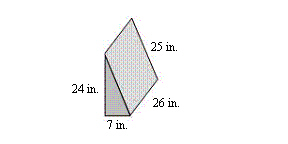Draw a solid with the following front, side, and top views.

1.

front: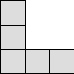side: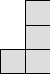top: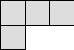2.

The floor makes up 40% of the lateral surface area of the tent. What is the value of x?